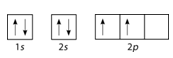5.17: Electron Configurations

If you keep your papers in manila folders, you can pick up a folder and see how much it weighs. If you want to know how many different papers (articles, bank records, or whatever else you keep in a folder), you have to take everything out and count. A computer directory, on the other hand, tells you exactly how much you have in each file. We can get the same information on atoms. If we use an orbital filling diagram, we have to count arrows. When we look at electron configuration data, we simply add up the numbers.

Electron Configurations

Electron configuration notation eliminates the boxes and arrows of orbital filling diagrams. Each occupied sublevel designation is written followed by a superscript that is the number of electrons in that sublevel. For example, the hydrogen configuration is $$1s^1$$, while the helium configuration is $$1s^2$$. Multiple occupied sublevels are written one after another. The electron configuration of lithium is $$1s^2 2s^1$$. The sum of the superscripts in an electron configuration is equal to the number of electrons in that atom, which is in turn equal to its atomic number.

Example $$\PageIndex{1}$$

Draw the orbital filling diagram for carbon and write its electron configuration.

Solution:

Step 1: List the known quantities and plan the problem.

Known

• Atomic number of carbon, $$Z=6$$

Use the order of fill diagram to draw an orbital filling diagram with a total of six electrons. Follow Hund's rule. Write the electron configuration.

Step 2: Construct DiagramOrbital filling diagram for carbon.

Electron configuration $$1s^2 2s^2 2p^2$$

Following the $$2s$$ sublevel is the $$2p$$, and $$p$$ sublevels always consist of three orbitals. All three orbitals need to be drawn even if one or more is unoccupied. According to Hund's rule, the sixth electron enters the second of those $$p$$ orbitals and with the same spin as the fifth electron.

Second Period Elements

Periods refer to the horizontal rows of the periodic table. Looking at a periodic table you will see that the first period contains only the elements hydrogen and helium. This is because the first principal energy level consists of only the $$s$$ sublevel and so only two electrons are required in order to fill the entire principal energy level. Each time a new principal energy level begins, as with the third element lithium, a new period is started on the periodic table. As one moves across the second period, electrons are successively added. With beryllium $$\left( Z=4 \right)$$, the $$2s$$ sublevel is complete and the $$2p$$ sublevel begins with boron $$\left( Z=5 \right)$$. Since there are three $$2p$$ orbitals and each orbital holds two electrons, the $$2p$$ sublevel is filled after six elements. Table $$\PageIndex{1}$$ shows the electron configurations of the elements in the second period.

Element Name Symbol Atomic Number Electron Configuration
Table $$\PageIndex{1}$$: Electron Configurations of Second-Period Elements
Lithium $$\ce{Li}$$ 3 $$1s^2 2s^1$$
Beryllium $$\ce{Be}$$ 4 $$1s^2 2s^2$$
Boron $$\ce{B}$$ 5 $$1s^2 2s^2 2p^1$$
Carbon $$\ce{C}$$ 6 $$1s^2 2s^2 2p^2$$
Nitrogen $$\ce{N}$$ 7 $$1s^2 2s^2 2p^3$$
Oxygen $$\ce{O}$$ 8 $$1s^2 2s^2 2p^4$$
Fluorine $$\ce{F}$$ 9 $$1s^2 2s^2 2p^5$$
Neon $$\ce{Ne}$$ 10 $$1s^2 2s^2 2p^6$$

Summary

Electron configuration notation simplifies the indication of where electrons are located in a specific atom. Superscripts are used to indicate the number of electrons in a given sublevel.

Contributors

• CK-12 Foundation by Sharon Bewick, Richard Parsons, Therese Forsythe, Shonna Robinson, and Jean Dupon.